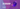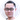Share:

## The Weird Parts Of JavaScript

My first experience with code was during National Service when I purchased a book on Python and wrote my first “Hello World!” file. Since then, I have progressed to pick up other programming languages such as JavaScript, Ruby and Java. The following is a summary of the quirks and odd features of JavaScript that I have came across in the last year.

### The typeof operator

The typeof operator returns a string stating the type of the input value.

`typeof 'hello world!'; // 'string'`
`typeof 123; // 'number'`
`typeof undefined; // 'undefined'`
`typeof true; // 'boolean'`
`typeof { a: 1, b: 2, c: 3 }; // 'object'`
```function sayHello() {
console.log('Hello world');
}```
`typeof sayHello; // 'function'`

There is no ‘array’ type in JavaScript, use Array.isArray() to check for an array

`typeof [1, 2, 3, 4, 5]; // 'object'`
`Array.isArray([1, 2, 3, 4, 5]); // true`

Performing mathematical operations on non-numbers results in NaN (not a number)

Somehow NaN (not a number) is a number?

```const foo = 5 / 'hello'; // NaN
typeof foo; // 'number'```

Ever heard the saying “Everything in JavaScript is an object”?

`typeof null; // 'object'`

### More on NaN

Trying to evaluate NaN to be equal to anything will result in false.

`51 === NaN; // false`
`'hello' === NaN; // false`
`['this', 'is', 'an', 'array'] === NaN; // false`
`null === NaN; // false`

Evaluating NaN with NaN results in false too.

`NaN == NaN; // false`
`NaN === NaN; // false`
```const notANumber = 'abc' - 100; // NaN
notANumber === NaN; // false```

We can check for NaN using the built in isNaN() function. It converts the input value to type Number before returning true for a NaN value.

```const notANumber = 'abc' - 100; // NaN
isNaN(notANumber); // true```
```isNaN('hello world'); // true
isNaN('12345'); // false - Number('12345') returns 12345```

### Implicit Coercion

Explicit coercion is an obvious attempt from the author to convert a value of one type to another type.

```const str = '12345';
typeof str; // 'string'```
```const num1 = parseInt(str);
const num2 = Number(str);```
```typeof num1; // 'number'
typeof num2; // 'number'```

Implicit coercion can be unclear and may be an unintended side effect.

Are these strings or numbers?

```const str = '12345';
typeof str; // 'string'```
```// Using the + operator
const plus = +str;
typeof plus; // 'number'```
```// String representation of a Number * 1
const times = str * 1;
typeof plus; // 'number'```

Using !! to get a Boolean value of the input, indicating if it is truthy or falsy.

```const zero = 0;
const one = 1;```
```!!zero; // false
!!one; // true```
```const str = 'Hi this is a string.';
const emptyStr = '';```
```!!str; // true
!!emptyStr; // false```

Here are some more examples of implicit coercion that gets confusing.

```!![]; // true
+[]; // 0
+!+[]; // 1
!+[] + !+[]; // 2
[+!+[]] + [+[]]; // '10'
[][[]]; // undefined
+[![]]; // NaN```
```typeof [] + []; // 'string'
typeof +[]; // 'number'
typeof ![]; // 'boolean'```

### Scope & hoisting

Variables declared with var are function scoped.

```function someFunction() {
for (var i = 0; i < 5; i++) {
console.log(`Inside the loop, i is \${i}`);
}```
```console.log(`Out of the loop, i is \${i}`);
}```
```someFunction();
// 'Inside the loop, i is 0'
// 'Inside the loop, i is 1'
// 'Inside the loop, i is 2'
// 'Inside the loop, i is 3'
// 'Inside the loop, i is 4'
// 'Out of the loop, i is 5'```

Variables declared with let are block scoped.

```function anotherFunction() {
for (let i = 0; i < 5; i++) {
console.log(`Inside the loop, i is \${i}`);
}```
```console.log(`Out of the loop, i is \${i}`);
}```
```anotherFunction();
// 'Inside the loop, i is 0'
// 'Inside the loop, i is 1'
// 'Inside the loop, i is 2'
// 'Inside the loop, i is 3'
// 'Inside the loop, i is 4'
// 'ReferenceError: i is not defined'```

Function declarations are hoisted to the top of the file, they can be called before they are declared. On the other hand, function expressions are not hoisted, they cannot be called before they are expressed.

```helloDeclaration(); // 'hello function declaration'
// Declaration
function helloDeclaration() {
console.log('hello function declaration');
}```
```helloExpression(); // TypeError: helloExpression is not a function
// Expression
const helloExpression = function() {
console.log('hello function expression');
};```

Variable declarations are hoisted to the top of the file but the assignment of values are left in place for runtime execution.

`a = 2; // this line (assignment) is left to be executed during runtime, it runs later`
`var a; // this line (variable declaration) is hoisted to the top of the file, it runs first`
`console.log(`a is \${a}`); // 'a is 2'`

Comparing the above snippet with this:

`console.log(`a is \${a}`); // 'a is undefined'`
```var a = 2;
// this statement is broken into two parts: var a; and a = 2;
// var a (variable declaration) is hoisted to the top of the file, a = 2 (value assignment) is not hoisted```

### Strict equality vs loose equality

JavaScript has == and === to check for equality, as well as != and !== to check for non-equality.

=== is known as strict equality, it checks for both value and type equality. On the other hand, loose equality is represented by == and it only checks for value equality. Coercion is allowed for == and JavaScript will attempt to convert the values to a common type.

```const num = 123;
const str = '123';```
```num == str; // true
num === str; // false```
```1 == true; // true
1 === true; // false```
```0 == false; // true
0 === false; // false```

### Comparing arrays and objects

Arrays and objects are reference types. Comparing 2 different arrays/objects using == or === returns false as they point to different arrays/objects in memory. To compare the elements of an array and the key/value pairs of an object, a deep comparison has to be done.

```const arr1 = [1, 2, 3, 4, 5];
const arr2 = [1, 2, 3, 4, 5];```
```arr1 == arr2; // false
arr1 === arr2; // false```
```const obj1 = { a: 1, b: 2, c: 3 };
const obj2 = { a: 1, b: 2, c: 3 };```
```obj1 == obj2; // false
obj1 === obj2; // false```

### Infinity and -infinity

Number​.POSITIVE_INFINITY is a numeric value representing infinity, it can also be written as Infinity.

Number.NEGATIVE_INFINITY equates to negative infinity, it can also be written as -Infinity.

```Infinity + Infinity; // Infinity
Infinity - Infinity; // NaN
Infinity * Infinity; // Infinity
Infinity / Infinity; // NaN```
```-Infinity + -Infinity; // -Infinity
-Infinity - -Infinity; // NaN
-Infinity * -Infinity; // Infinity
-Infinity / -Infinity; // NaN```

The built in Math object includes helpful methods such as Math.max() and Math.min(), they return the input maximum and minimum values, respectively.

```Math.max(1, 20, 300, 4000, 50000); // 50000
Math.min(-1, -20, -300, -4000, -50000); // -50000```

What happens if no arguments are passed into Math.max() and Math.min()?

```Math.max(); // -Infinity
Math.min(); // Infinity```

It has been a quirky yet enjoyable experience over the past year and I look forward to learning more JavaScript 🙃Jay Lee
Curious thinker, problem solver; Jay drinks coffee and writes code.